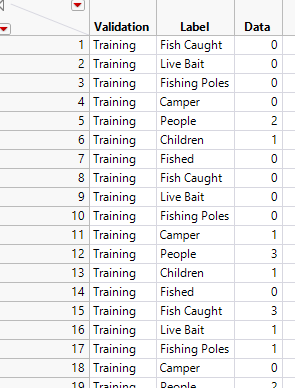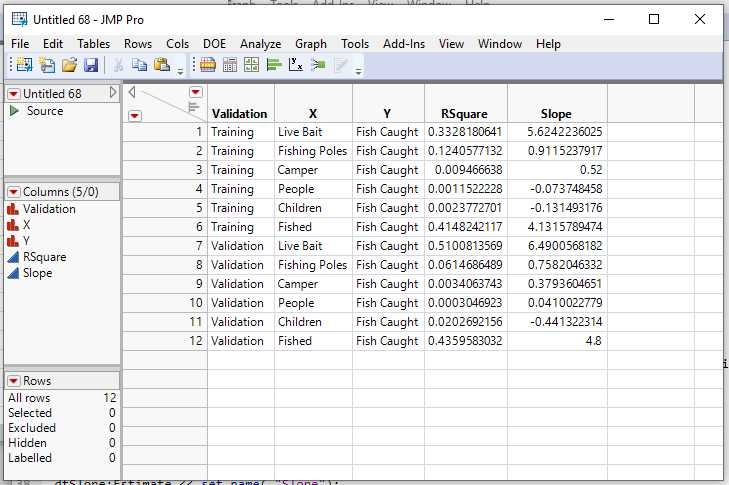Choose Language Hide Translation Bar
Highlighted

## How to group by and get slopes in a JSL fit model script

Hi,

I'm trying to fit data in group and get slope and R squared number. I'm using Fishing data set as an example here.

I stacked the data so that the summary will be outputted easily as one single column then I need to spread the columns again.

So I did

``````fishing_stacked = dt << Stack(
columns(
:Fish Caught,
:Live Bait,
:Fishing Poles,
:Camper,
:People,
:Children,
:Fished
),
Source Label Column( "Label" ),
Stacked Data Column( "Data" )
);``````I want to group the data by `Validation` column and fit (Y axis = Fish cought )and X axis are "Live Bait, Fishing Poles, Camper ...." and get the slope value and R squared number as new columns.

Here is the previous post but I don't know how to apply it to my data set.

https://community.jmp.com/t5/Discussions/Save-Parameter-Estimates-of-Linear-Regression-with-by-Varia...

2 ACCEPTED SOLUTIONS

Accepted Solutions
Highlighted

## Re: How to group by and get slopes in a JSL fit model script

1. The analysis can not be directly done from the stacked data table.  In order to perform the bivariates, the Fish Caught needs to be in a separate column, and paired with each of the X Factors.  However, I think what you may want is just the output format,and this script produces it from the original data table

``````NamesDefaultToHere(1);

dt = Open("\$SAMPLE_DATA/Fishing.jmp");

biv = Bivariate(invisible,
Y( :Fish Caught ),
X( :Live Bait,
:Fishing Poles,
:Camper,
:People,
:Children,
:Fished),
Fit Line( {Line Color( "Medium Dark Red" )} ),
By( :Validation )
);

// Create the data table of RSqares
dtR2 = report(biv)["Summary of Fit"][tablebox(1)]<< make combined data table;
dtSlope = report(biv)["Parameter Estimates"][tablebox(1)]<< make combined data table(invisible);
dtR2 << select where(:Column 1 != "RSquare");
dtR2 << delete rows;
dtR2:Column 2 << set name("RSquare");
dtR2 << delete Columns({"Validation 2","Column 1"});

// Create the data table of Slopes
dtSlope << select where(:Term == "Intercept");
dtSlope << delete rows;
dtSlope:Estimate << set name( "Slope");
dtSlope << delete columns({"Validation 2","Term","~Bias","Std Error","t Ratio","Prob>|t|"});

// Put the data together
dtR2 << Update(
With( dtSlope ),
Match Columns( :Validation = :Validation, :X = :X, :Y = :Y )
);

// Clean up the items not needed
close(dtSlope, nosave);
window("Fishing - Bivariate of Fish Caught") << close window;``````
Jim
Highlighted

## Re: How to group by and get slopes in a JSL fit model script

I would approach this differently.  By placing all of the output into a single window, the

<< Make Combined Data Table

will work as desired.  Working across separate windows will be an issue.

I choose to modify the list of x columns one wants to use, and then apply it to only one Bivariate execution

``````Names Default To Here( 1 );

dt = Open( "\$SAMPLE_DATA/Fishing.jmp" );

colList = dt << get column names( string );

For( i = N Items( colList ), I >= 1, i--,
If( Contains( colList[i], "Validation" ) | Contains( colList[i], "People" ),
Remove From( colList, i, 1 )
)
);

biv = Bivariate(invisible,
Y( :Fish Caught ),
X( eval(colList) ),
Fit Line( {Line Color( "Medium Dark Red" )} ),
By( :Validation )
);

// Create the data table of RSqares
dtR2 = report(biv)["Summary of Fit"][tablebox(1)]<< make combined data table;
dtSlope = report(biv)["Parameter Estimates"][tablebox(1)]<< make combined data table (invisible);
dtR2 << select where(:Column 1 != "RSquare");
dtR2 << delete rows;
dtR2:Column 2 << set name("RSquare");
dtR2 << delete Columns({"Validation 2","Column 1"});``````

There is also a Platform that you may want to explore, that directly produces the report you want.

``````Names Default To Here( 1 );

dt = Open( "\$SAMPLE_DATA/Fishing.jmp" );

colList = dt << get column names( string );

For( i = N Items( colList ), I >= 1, i--,
If( Contains( colList[i], "Validation" ) | Contains( colList[i], "People" ) |
Contains( colList[i], "Fish Caught" ),
Remove From( colList, i, 1 )
)
);

Response Screening(
Y( :Fish Caught ),
X( eval(colList) ),
Where( Format( :Validation ) == "Training" )
);``````

Jim
13 REPLIES 13
Highlighted

## Re: How to group by and get slopes in a JSL fit model script

any solution to this ?
Highlighted

## Re: How to group by and get slopes in a JSL fit model script

is it so difficult to do this ?
Highlighted

## Re: How to group by and get slopes in a JSL fit model script

I am not sure that stacking will help, given the analysis you intend. I would try selecting Analyze > Fit Y by X. Select Fish Caught and click Y. Select the other columns and click X. Select Validation and click By, then click OK. Hold the Control key (Windows) or Command key (Macintosh), click the red triangle next to Bivariate and select Fit Line. Right-click the table in Summary of Fit and select Make Into Combined Table. Right-click the table in Parameter Estimates and select Make Into Combined Table.

Learn it once, use it forever!
Highlighted

## Re: How to group by and get slopes in a JSL fit model script

Can we do this as JSL script ?
Highlighted

## Re: How to group by and get slopes in a JSL fit model script

``````NamesDefaultToHere(1);

dt = Open("\$SAMPLE_DATA/Fishing.jmp");
xList = {:Fishing Poles, :People, :Children};
biv = dt << Bivariate(Y(:Fish Caught), X(Eval(xList)), By(:Validation), FitLine(1));``````
Highlighted

## Re: How to group by and get slopes in a JSL fit model script

thanks but how can I get slope and R squared number extracted from Bivariate plots ? In addition, can we do this in stacked version of the Fish data as showed in table ?
Highlighted

## Re: How to group by and get slopes in a JSL fit model script

1. The analysis can not be directly done from the stacked data table.  In order to perform the bivariates, the Fish Caught needs to be in a separate column, and paired with each of the X Factors.  However, I think what you may want is just the output format,and this script produces it from the original data table

``````NamesDefaultToHere(1);

dt = Open("\$SAMPLE_DATA/Fishing.jmp");

biv = Bivariate(invisible,
Y( :Fish Caught ),
X( :Live Bait,
:Fishing Poles,
:Camper,
:People,
:Children,
:Fished),
Fit Line( {Line Color( "Medium Dark Red" )} ),
By( :Validation )
);

// Create the data table of RSqares
dtR2 = report(biv)["Summary of Fit"][tablebox(1)]<< make combined data table;
dtSlope = report(biv)["Parameter Estimates"][tablebox(1)]<< make combined data table(invisible);
dtR2 << select where(:Column 1 != "RSquare");
dtR2 << delete rows;
dtR2:Column 2 << set name("RSquare");
dtR2 << delete Columns({"Validation 2","Column 1"});

// Create the data table of Slopes
dtSlope << select where(:Term == "Intercept");
dtSlope << delete rows;
dtSlope:Estimate << set name( "Slope");
dtSlope << delete columns({"Validation 2","Term","~Bias","Std Error","t Ratio","Prob>|t|"});

// Put the data together
dtR2 << Update(
With( dtSlope ),
Match Columns( :Validation = :Validation, :X = :X, :Y = :Y )
);

// Clean up the items not needed
close(dtSlope, nosave);
window("Fishing - Bivariate of Fish Caught") << close window;``````
Jim
Highlighted

## Re: How to group by and get slopes in a JSL fit model script

JMP has no out of the box calculation module for slope?
Highlighted

## Re: How to group by and get slopes in a JSL fit model script

Sure, it does! You can use either the Bivariate platform or the Fit Least Squares platform to estimate the slope in the simple linear regression model. There is also a Linear Regression() function if you need such estimates in a formula or a script.

Learn it once, use it forever!
Article Labels

There are no labels assigned to this post.